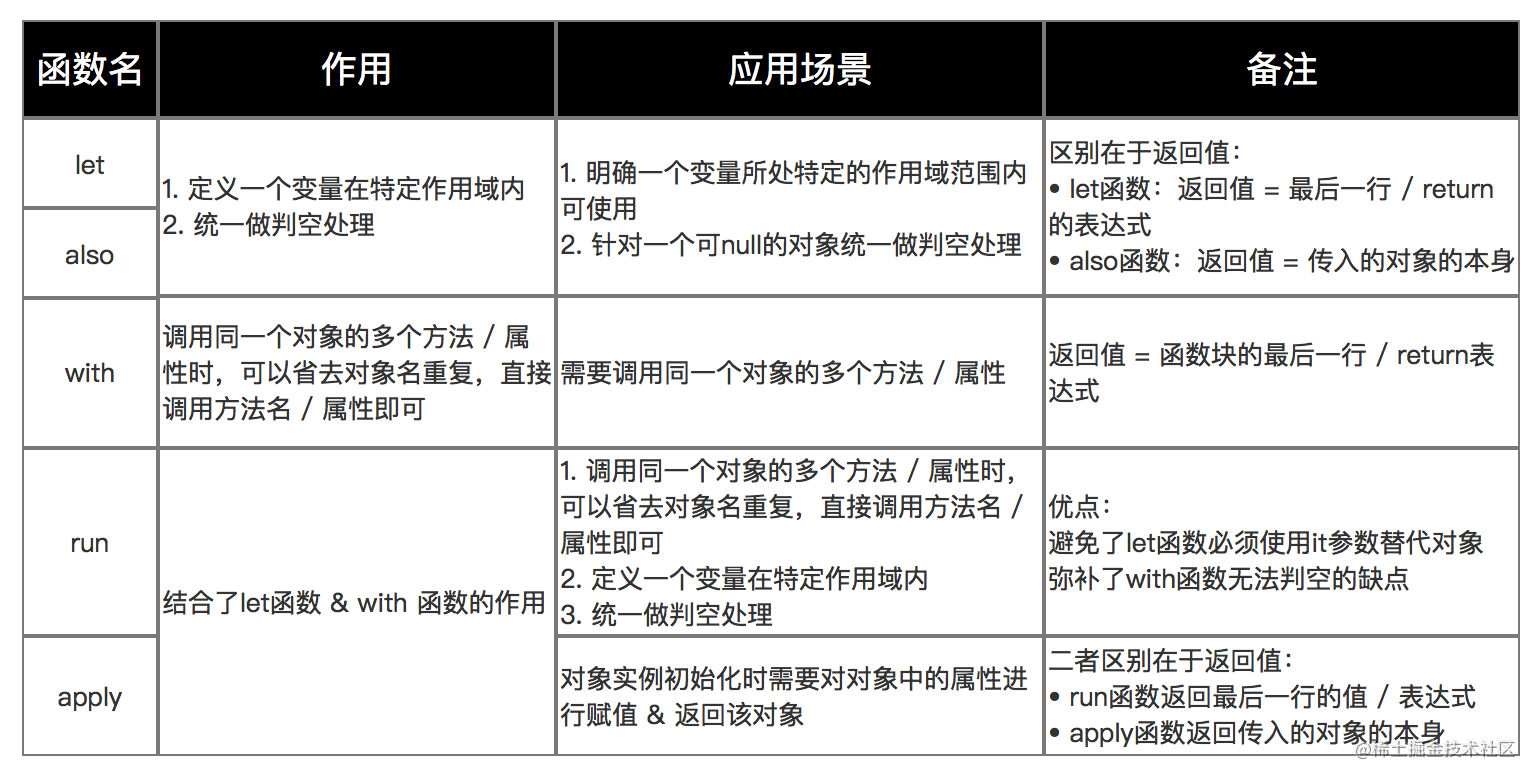# Kotlin 高阶函数: let/also/with/run/apply

## let

### 函数签名

``````@kotlin.internal.InlineOnly
public inline fun <T, R> T.let(block: (T) -> R): R {
contract {
callsInPlace(block, InvocationKind.EXACTLY_ONCE)
}
return block(this)
}

### 使用场景

1. 针对一个可 `null` 对象统一做判空处理，避免写一些判断 `null` 的操作
``````// 如果 object 为 null，则 let 闭包中的方法不会被执行
// 如果 object 不为 null，则 let 闭包中默认的 it 变量为不可选类型
object?.let {
it.doSomething()
// 闭包的返回值
"xxx"
}

1. 使用 `it` 替代 `object` 对象去访问公有的属性 & 方法
``````// 无论 object 是否为空均会执行，则 let 闭包中默认的 it 变量和 object 保持一致
object.let {
it.doSomething()
// 闭包的返回值
"xxx"
}

### 使用示例

``````block?.let { it() }

## also

### 函数签名

``````public inline fun <T> T.also(block: (T) -> Unit): T {
contract {
callsInPlace(block, InvocationKind.EXACTLY_ONCE)
}
block(this)
// 闭包的返回值为 this
return this
}

### 和 let 区别

• `let`: 返回值为最后一行
• `also`: 返回值为 `this`

### 使用场景

``````val object = Object().also {
it.xxx = "xxx"
it.doSomething()
}

## with 函数

### 函数签名

``````public inline fun <T, R> with(receiver: T, block: T.() -> R): R {
contract {
callsInPlace(block, InvocationKind.EXACTLY_ONCE)
}
}

### 使用场景

``````// 闭包里面的变量为 this，而不是 it
with(object) {
// 这里就相当于 object.doSomething()
doSomething()
// 闭包的返回值
xxx
}

## run

### 函数签名

``````public inline fun <T, R> T.run(block: T.() -> R): R {
contract {
callsInPlace(block, InvocationKind.EXACTLY_ONCE)
}
return block()
}

### 作用

1. 可以判空
2. 直接调用，无需 `it`

### 使用场景

``````object?.run {
doSomething()
}

## apply

### 函数签名

``````public inline fun <T> T.apply(block: T.() -> Unit): T {
contract {
callsInPlace(block, InvocationKind.EXACTLY_ONCE)
}
block()
return this
}

### 和 run 区别

1. `run` 的返回值为闭包的最后一行
2. `apply` 的返回值为 `this`

### 使用场景

``````class Test {
fun test() = apply {
doSomething()
}

fun test1() = apply {
doSomething()
}
}

// other class
Test().run {
test().test1()
}

## 总结

### 如果抉择

`let/run` 由于返回值为最后一行，更适用于一次执行。

`also/apply` 由于返回值为 `this`，更适用于链式调用。

### 特殊点

`(T) -> R` 可以看成是把 `this` 当成参数传入闭包中进行处理。

`T.() -> R` 可以看成是给 `T` 类型的类扩展了一个 `() -> R` 的属性，当 `receiver.block()` 被执行的时候，相当于 `T` 类型中扩展的 `() -> R` 类型的闭包被执行了。Android

Android